Question

# Write a C++ document program

Write a C++ document program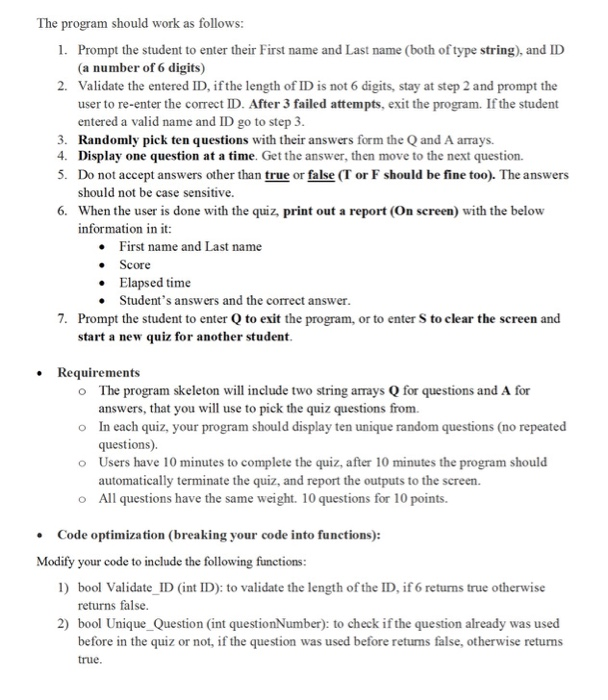questions.txt
Where does a C++ program begin is execution?
The first function in the file
The first function by alphabetic order of names
The function main( )
The function specified in the #DEFINE FirstFunction

How many values can be returned from a function by means of the "return" statement?
1
2
3
Lots and Lots
Which loop type is guaranteed to execute at least once?
for
while
do...while
all the above
Arrays are passed to functions by:
reference
value
either method
neither method
Global variables should be used...
in place of function parameters
only when absolutely needed
never
only during the full moon
When storing a floating point value to an integer type variable, the fractional part is:
rounded up
rounded down
branched
truncated
C
A
C
A

B
d
main.cpp
#include <iostream>
#include <fstream>
#include <string>
#include <cctype>
#include <ctime>
#include <cstdlib>
using namespace std;

int play_game(int , char [], string [], string);
void show_question(string [], int, char);
char player_try();
void sort_score(string, int);

/*=====================================================================
Function: main
Description: A high level organizer. Check the command line arguments, calls
the functions to read the input files, check that the questions and answer
files have the same number of items, call the play_game( ) function, call
sort_score( ) function.
Parameters: int argc - argument count
char *argv[] - hold arguments
======================================================================*/
int main(int argc, char *argv[])
{
if (argc != 3)
{
cout << "Command line arguments are incorrect." << endl;
return -1;
}
ifstream fin_q(argv);
//If questions file does not exist. Terminate.
if(!fin_q)
{
cout << "Questions file failed to open. Program terminated.";
return -1;
}
ifstream fin_ans(argv);
//If answers file does not exist. Terminate.
if(!fin_ans)
{
cout << "Answers file failed to open. Program terminated.";
return -1;
}

//Create array to hold questions and enter read_questions function
string questions;
// If read_questions returns "-1" display error and terminate.
if(qCount == -1)
{
cout << "Error: Questions file empty.";
return -1;
}
// If # of answers does not equal # of questions display error & terminate.
if(qCount != count2)
{
cout << "Error: Number of answers does not match number of questions. ";
return -1;
}

// Get player name.
string name;
cout << "Please enter player name: ";
cin >> name;
// Main play_game function, return final_score.
int final_score = play_game( qCount, answers, questions, name );
cout << endl << "Final score: " << final_score << endl;

// Create and open summary.txt.
string fname = "summary";
fname+= ".txt";
ofstream fout(fname.c_str(), ios:: app);
if(!fout)
{
cout << "Cannot write to the target file." << endl;
return -1;
}
//Write name and score to summary.txt
fout << name << " " << final_score << endl;

//Enter sort_score function to get top score and player rank.
sort_score(name, final_score);

cout << "GG." << endl <<endl;

// Close all files before exit.
fin_q.close();
fin_ans.close();
fout.close();
return 0;
}

/*=====================================================================
Description: Function reads questions.txt into a 2d array of strings and
returns the number of questions
Parameters: ifstream &fin_q   - file to read the questions from
string questions[] - array to hold questions
======================================================================*/
{
int i = 0;       //counts number of questions
string test;   //temporary string to hold text from file

// If questions file empty return error to main.
if(!getline(fin_q,test))
{
return -1;
}
//While not end of file read questions to array
while(!fin_q.eof())
{
if(test.size() > 1)       //If text detected then begin loop
{
for(int j = 0; j < 5; j++)
{
questions[i][j] = test;
getline(fin_q, test);
}
i++;
}
getline(fin_q,test);
}
return i;
}

/*=====================================================================
======================================================================*/
{
int i = 0;       //loops through array and returns # of answers
char test;       //temporary char to hold text from file
while(!fin_ans.eof())
{
fin_ans >> test;
i++;
}
return i - 1;
}

/*=====================================================================
Function: play_game
Description: Main control of game play. Function picks questions, checks
correctness of player response, offers second chance, determines
and applies scoring. Returns final score.
Parameters: int qCount   - number of questions
string questions[] - array of questions and choices
string name - players name
======================================================================*/
int play_game(int qCount, char answers[], string questions[], string name )
{
bool gameON = 0;   //Flag that checks if unanswered questions remain
bool skip;           //Flag if player has skipped a question
char choice;       //Contains players choice
char chance;       //Flag if player has taken second chance
int qNum = 1;       //Contains the current round number
int score = 1;       //Contains and modifies player score
// Create array of bool and initialize to false.
bool check;
for(int i = 0; i < qCount; i++)
check[i] = false;

do
{
skip = 0;
chance = 'N';
choice = '0';
gameON = 0;
// Generate random question not used previously.
int rand_num;
srand((unsigned)time(NULL));
do
{
rand_num = rand( ) % qCount;
}while(check[rand_num] == 1);
check[rand_num] = 1;
// Display question.
cout << endl << name << " here's question number " << qNum;
show_question(questions, rand_num, '0');
// Ask for and accept player's first try.
choice = player_try();
// If correct answer. Give full points.
{
score *= 10;
cout << "Correct! Current score is " << score << endl;
}
else
{
cout << "Incorrect. "
"Second chance (Y) or (N)? > ";
cin >> chance;
chance = toupper(chance);
// Verify input is correct.
while(!((chance == 'Y') || (chance == 'N')))
{
cout << "Incorrect input. (Y) for second chance or (N) "
"to skip. ";
cin >> chance;
chance = toupper(chance);
}
cout << chance << endl;
// If select to skip question, keep score unchanged.
if(chance == 'N')
{
cout << "Question skipped. Score is " << score << endl;
}
// Else if select second chance, re-display question.
else if(chance == 'Y')
{
cout << endl << "Second chance.";
show_question(questions, rand_num, choice);
choice = player_try();
// If correct answer increase score by half points.
{
chance = 'N';
score *= 5;
cout << "Correct! Score is " << score <<endl;
}
// If wrong answer set score to 0 and announce Game Over.
else
{
score = 0;
cout << "Incorrect. GAME OVER!" << endl;
}
}
}
qNum++;
// If check array still has unanswered questions, continue game.
for(int i = 0; i < qCount; i++)
{
if(check[i] == 0)
gameON = 1;
}
}while((gameON == 1) && (chance == 'N'));

return score;
}

/*=====================================================================
Function: show_question
Description: Displays question and its choices to player.
Parameters: string questions[] - array of questions and choices
int rand_num - random question from array
char choice - previous choice user has made (A,B,C,D or none)
======================================================================*/
void show_question(string questions[], int rand_num, char choice)
{
//Display question.
cout << endl << questions[rand_num] << endl;
//Display appropriate responses based off previous choice (A-D).
switch(choice)
{
case '0':
cout << "A. " << questions[rand_num] << endl;
cout << "B. " << questions[rand_num] << endl;
cout << "C. " << questions[rand_num] << endl;
cout << "D. " << questions[rand_num] << endl;
break;
case 'A':
cout << "B. " << questions[rand_num] << endl;
cout << "C. " << questions[rand_num] << endl;
cout << "D. " << questions[rand_num] << endl;
break;
case 'B':
cout << "A. " << questions[rand_num] << endl;
cout << "C. " << questions[rand_num] << endl;
cout << "D. " << questions[rand_num] << endl;
break;
case 'C':
cout << "A. " << questions[rand_num] << endl;
cout << "B. " << questions[rand_num] << endl;
cout << "D. " << questions[rand_num] << endl;
break;
case 'D':
cout << "A. " << questions[rand_num] << endl;
cout << "B. " << questions[rand_num] << endl;
cout << "C. " << questions[rand_num] << endl;
break;
}
}

/*=====================================================================
Function: player_try
Description: get the choice from player, validate response is in range A-D,
return players choice.
Parameters: none
======================================================================*/
char player_try()
{
//Ask for and hold player choice
char choice;
cout << "Your choice? > ";
cin >> choice;

// Verify that choice is in range A-D, else print out warning
switch(choice)
{
case 'a':
case 'b':
case 'c':
case 'd':
choice = toupper(choice);
break;
case 'A':
case 'B':
case 'C':
case 'D': break;
default:
cout << "Invalid selection. Please enter A, B, C, or D." << endl;
player_try();
break;
}
return choice;
}

/*=====================================================================
Function: sort_score
Description: read in records of previous players. Sort the scores and names,
display the current high score and players name, and display the rank of
the current player based on his/her score.
Parameters: string name - players name
int final_score - final score of current player
======================================================================*/
void sort_score(string name, int final_score)
{
int score;       //array of arbitrary length to hold scores
string player;   //array of arbitrary length to hold names
int count = 0;       //counts number of records in summary.txt
//open and verify summary.txt
ifstream fin_scores("summary.txt");
if(!fin_scores)
cout << "Could not open summary.txt";

//while not end of file read in names and scores from file to arrays
while(!fin_scores.eof())
{
fin_scores >> player[count];
fin_scores >> score[count];
count++;
}
int total_records = count-1;   //total # records in summary.txt

//selection sort records from highest to lowest.
int startScan, maxIndex, maxValue;
string maxPlayer;
for (startScan = 0; startScan < (total_records - 1); startScan++)
{
maxIndex = startScan;
maxValue = score[startScan];
for(int index = startScan + 1; index < total_records; index++)
{
if (score[index] > maxValue)
{
maxValue = score[index];
maxPlayer = player[index];
maxIndex = index;
}
}
//assign player and score to correct array position
score[maxIndex] = score[startScan];
player[maxIndex] = player[startScan];
score[startScan] = maxValue;
player[startScan] = maxPlayer;
}

//display current high score
cout << endl << "===================================" << endl;
cout << "Current high score: " << player << " " << score << endl;
//find and display current rank of player
for(int i = 0; i < total_records; i++)
{
if(player[i] == name && score[i] == final_score)
{
cout << "You ranked #" << i+1 << " of "
<< total_records << endl;
break;
}
}
//close fin_scores file
fin_scores.close();
}
summary.txt
second 10000000
THIRD 0
fourth 5000
1
1
a 1

#### Earn Coins

Coins can be redeemed for fabulous gifts.

Similar Homework Help Questions
• ### reword m the program into a design document diregard the m Write a C++ program that...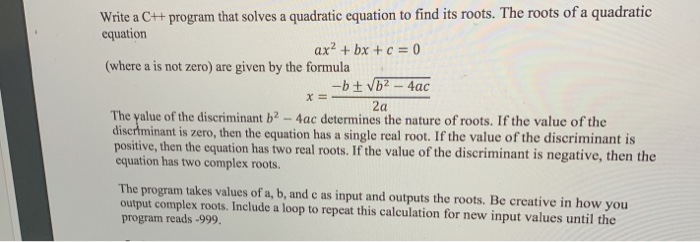reword m the program into a design document diregard the m Write a C++ program that solves a quadratic equation to find its roots. The roots of a quadratic equation ax2 + bx + c = 0 (where a is not zero) are given by the formula -b + b2 - 4ac 2a The value of the discriminant b2 - 4ac determines the nature of roots. If the value of the discriminant is zero, then the equation has a single...

• ### Write a Java program to calculate the fee for photo-coping document. The program will read an...

Write a Java program to calculate the fee for photo-coping document. The program will read an integer number from the keyboard which is the number of photocopies to be produced. The program will also calculate the fee as follow: - if the number of copies is less than 100, each copy costs \$0.10 - if the number of copies is between 100 and 499 (inclusive), each copy costs \$0.05 - if the number of copies is between 500 and 999...

• ### Write a Matlab Program to perform recovery of original document from torn fragments using Image Mosaicing...

Write a Matlab Program to perform recovery of original document from torn fragments using Image Mosaicing of binary Image Processing with two step approach. Initially identify the initial set of matching fragment pairs and resolve the ambiguity among these fragments by reconstructing in to a original document

• ### Program description: Write the necessary C++ statements (Program) to calculate the employee's weekly pay. Where :...

Program description: Write the necessary C++ statements (Program) to calculate the employee's weekly pay. Where : Hour = employee's work hours Rate = employee's hourly pay Wages = employee's weekly pay Given that ( Hour= 31.5 and , Rate = \$11.45) Write the variable declarations and initialization sections necessary to compute and print the required calculations. The program should ask the user to input the hours and Rate of pay, then calculate the weekly Wages. Hint: Modify program in project...

• ### Attached to this assignment as a separate document is the C++ code for a program that...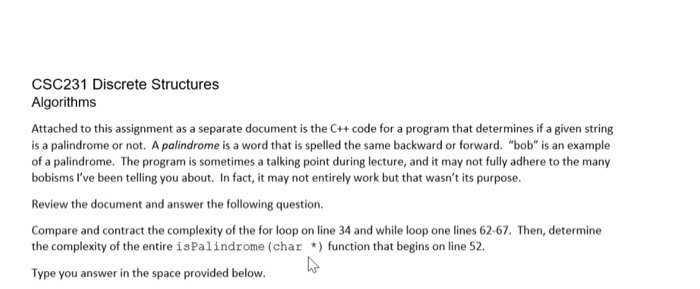Attached to this assignment as a separate document is the C++ code for a program that determines if a given string is a palindrome or not. A palindrome is a word that is spelled the same backward or forward. "bob" is an example of a palindrome. The program is sometimes a talking point during lecture, and may not fully adhere to the many bobisms I've been telling you about. In fact, it may not entirely work but that wasn't its...

• ### For C++ Write a main program that inputs a list of 20 numbers from a text file and then calls 4 ...

For C++ Write a main program that inputs a list of 20 numbers from a text file and then calls 4 sort functions. Create a Sort Lab design document that include flowcharts of the main program and four sort functions Sort Functions:   Insertion, Selection, Bubble and Merge In each Sort function: count the number of compares and swaps needed to sort the numbers and display that information in the sort function. Run the program for four sets of data --...

• ### C++ Program Help. Was just wondering how to write such code to perform this. - Take...

C++ Program Help. Was just wondering how to write such code to perform this. - Take approximately 360 words from any English document as your input text. Ignore all blanks, all punctuation marks, all special symbols. Create an input file with this input text - Have the program to read the input from the input file "infile.dat".

• ### This is a C++ question. Hi , I would like to write a program that read...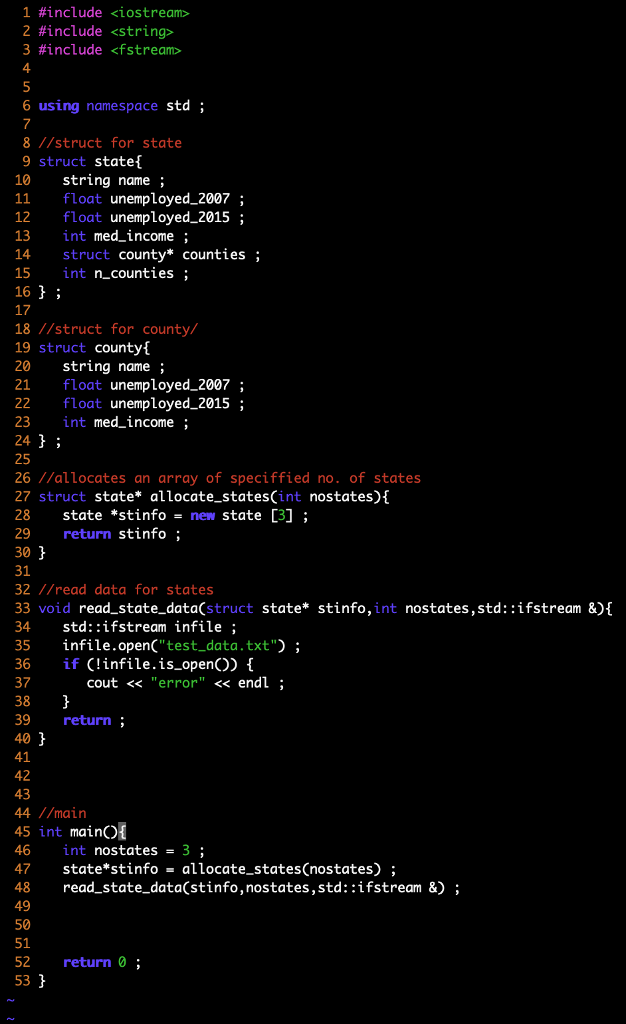This is a C++ question. Hi , I would like to write a program that read a file from a txt document. I have to create an arracy of type struct to contain the data. I have problem in using the function I have written. What should be the parameters when I want to call a function that read a file?

• ### CSC 211 - Lab-2 Write a C++ program to find the roots of a quadratic equation...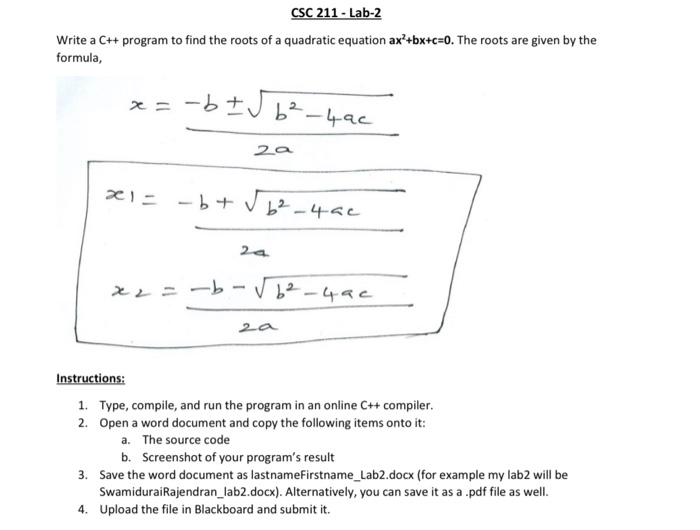CSC 211 - Lab-2 Write a C++ program to find the roots of a quadratic equation ax +bx+c=0. The roots are given by the formula, x=-b I56²-4ac 2a x = -b+ √b²-4ac 2. x2 = -b-√6²-4ac 2a Instructions: 1. Type, compile, and run the program in an online C++ compiler. 2. Open a word document and copy the following items onto it: a. The source code b. Screenshot of your program's result 3. Save the word document as lastnameFirstname_Lab2.docx (for...

• ### Write a C++ Program Write a program that prompts the user to input a number. The...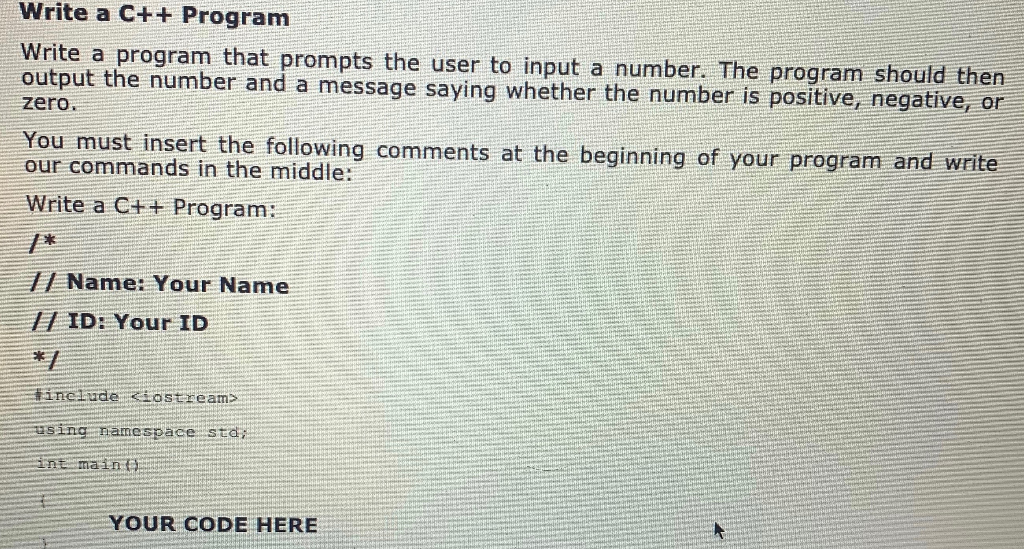Write a C++ Program Write a program that prompts the user to input a number. The program should then output the number and a message saying whether the number is positive, negative, or zero. You must insert the following comments at the beginning of your program and write our commands in the middle: Write a C++ Program: 1 Name: Your Name ID: Your ID #include <iostream> using namespace std; din main YOUR CODE HERE